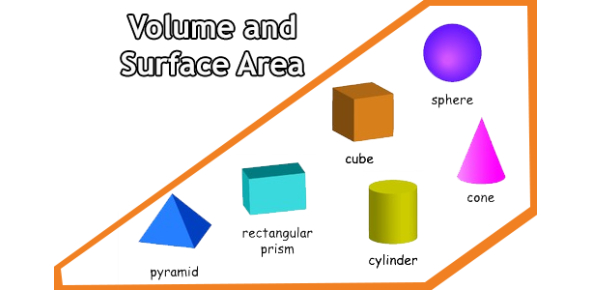# Volume And Surface Area Test! Quiz

8 Questions | Total Attempts: 76SettingsIf you are good at maths, then I believe you would not have a problem passing this volume and surface area test quiz! Volume is the amount of space that an object occupies, whereas the surface area is the uppermost layer of an object. Different shapes have different formulas for getting the area and surface area. In this quiz, you will get to test out just how well you use the procedures to answer all the questions. Do give it a shot and check out other tests like it to perfect your skills.

• 1.
9300 m = __________ km.
• A.

9.3 km

• B.

93 km

• C.

0.93 km

• D.

0.0093 km

• 2.
A regular polygon is a
• A.

Polygon with all equal sides and all same angles

• B.

Polygon with no equal sides and same angles.

• 3.
44.4 square kilometer equals
• A.

44400 ha

• B.

0.000444 ha

• C.

0.04444 ha

• D.

4440 ha

• 4.
4000 cubic milimeter
• A.

0.4 cubic centimeter

• B.

4 cubic centimeter

• C.

400 cubic centimeter

• D.

40 cubic centimeter

• 5.
58,000 cubic millimeter equals
• A.

58 cubic meter

• B.

0.058 cubic meter

• C.

0.0058 cubic meter

• D.

0.000058 cubic meter

• 6.
The volume of a prism = half of base area multiplied by the height.
• A.

True

• B.

False

• 7.
Surface area of prism = Perimeter of the base (height) + 2(base area)
• A.

True

• B.

False

• 8.
A Convex Polygon is a closed 2-D shape with no internal angles greater than 180o.
• A.

True

• B.

False

Related TopicsBack to top